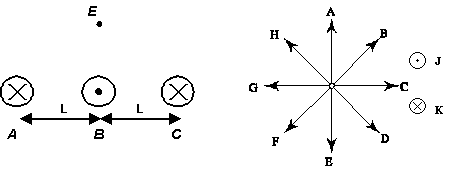Teaching Physics with the Physics Suite Edward F. Redish

Magnetic forces and fields

The figure at the left below shows a cross section of three long parallel wires (labeled A through C ) taken in a plane perpendicular to the wires. One or more of the wires may be carrying a current. If a wire carries a current it is in the direction indicated and has magnitude I0. For each of the five vector quantities (1) through (5) indicate the direction of the quantity on your answer using one of the letters associated with a directional arrow on the figure at the right below. If the magnitude of the quantity is zero, write "0". If the result is not 0 but points in a direction other than one of those indicated, write "N".1. the magnetic field at E if only wire A is carrying a current.
2. the magnetic field at wire C if only wire A is carrying a current.
3. the magnetic force on wire C if only wires A and C carry currents
4. the magnetic force on wire C if only wire A is carrying a current.
5. the magnetic force on a proton at E traveling to the right (i.e., in direction C) if only wire B is carrying a current.

Not finding what you wanted? Check the Site Map for more information.